Employees

Of all 360 employees, there are 11/12 women. How many men work in a company?

Result

m =  30

Solution:Leave us a comment of this math problem and its solution (i.e. if it is still somewhat unclear...):Be the first to comment!To solve this verbal math problem are needed these knowledge from mathematics:

Need help calculate sum, simplify or multiply fractions? Try our fraction calculator.

Next similar math problems:

1. PearsThere were pears in the basket, I took two-fifths of them, and left six in the basket. How many pears did I take?
2. Mountain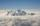Mountain has the elevation 7450 meters and in the morning is the middle portion thereof in the clouds. How many meters of height is in the sky if bellow the clouds is 2,000 meters and above clouds are two fifths of elevation of the mountain?
3. Coal mine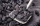The monthly plan of 17,000 tons of coal exceeded the mine by 1/25. How many tonnes of coal have been harvested from the mine above plan?
4. Package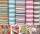The package was 23 meters of textile. The first day sold 12.3 meters. How many meters of textile remained in the package?
5. Equivalent fractionsAre these two fractions equivalent -4/9 and 11/15?
6. Watching TVOne evening 2/3 students watch TV. Of those students, 3/8 watched a reality show. Of the students that watched the show, 1/4 of them recorded it. What fraction of the students watched and recorded reality tv.
7. Classroom 4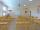In a class of 36 pupils, 2/3 are girls. How much it is in a class girls and boys?
8. EricaErica bought 3 1/2 yards of fabric. If she uses 2/3 of the fabric, how much will she have left?
9. Pie II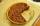Vili ate three pieces of pie. If it pieces is 1/8 how much pie did he eat?
10. Write 3Write a real world problem involving the multiplication of a fraction and a whole number with a product that is between 8 and 10 then solve the problem
11. Fractions 3Calculate 1/9 of 27:
12. KingKing had four sons. First inherit 1/2, second 1/4 , third 1/5 of property. What part of the property was left to the last of the brothers?
13. Bitoo and ReenaBitoo ate 3/5 part of an apple and the remaining part was eaten by his sister Reena. How much part of an apple did Renna eat? Who had the larger share? By how much?
14. Eq-fracSolve the following equation with fractions: h + 1/3 =5/3
15. One thirdIf 3/5 is 360, how much is 1/3?
16. EqnSolve equation with fractions: 2x/3-50=40+x/4
17. Write 2Write 791 thousandths as fraction in expanded form.